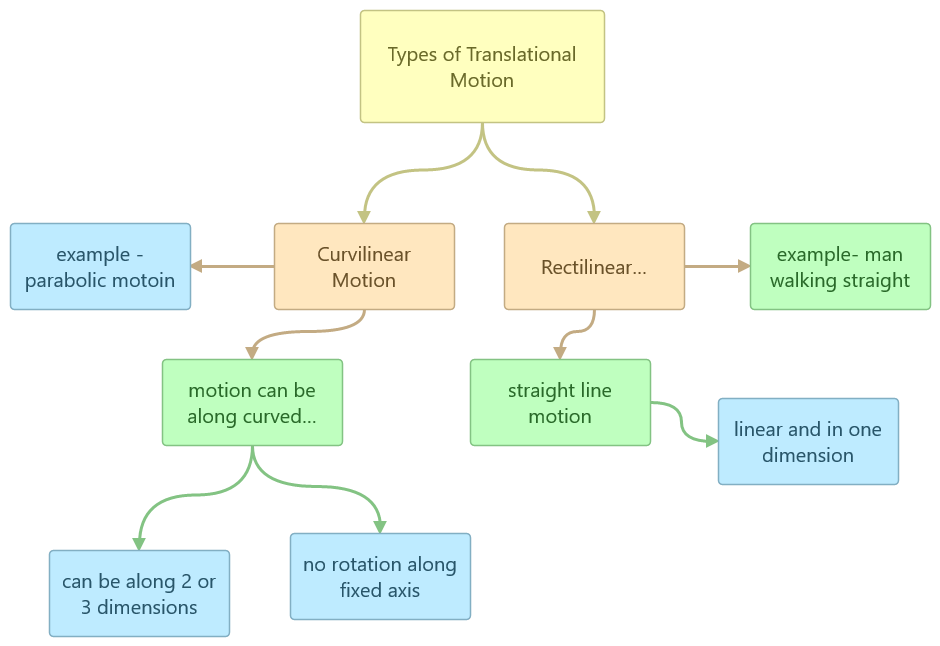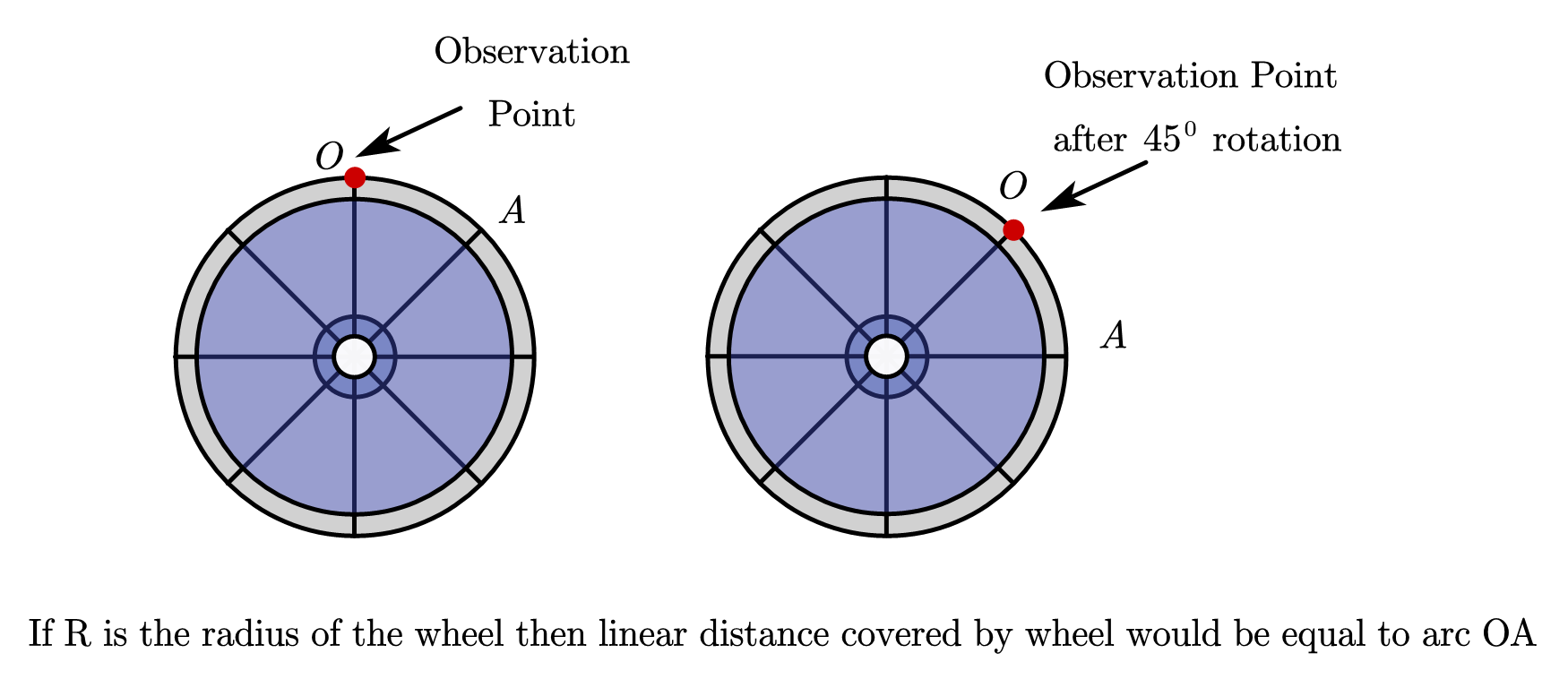# What is translational motion?

## Translational Motion

### Definition, Types of translational motion and examples

Motion in physics is defined as a change in position of an object with the passage of time.

### Translational motion definition

Translational motion is the motion in which all points of a moving body move uniformly in the same line or direction. If an object is executing translational motion then there is no change in its orientation relative to a fixed point. For example, a train moving in its track, a man walking on the road, birds flying in the sky, etc.

In this article, we are covering Translational motion with examples. If you want to learn about the basics of motion you can visit our article What is motion in physics to get a general overview of motion in physics

### What is translational motion?

When a body is shifted or moved from one point to another point, then the body said to be experienced translational motion. It is the motion in which all points of a moving body move uniformly in the same line or direction. If an object is executing translational motion then there is no change in its orientation relative to a fixed point

So,

If the object moves such that all the particles in the object move parallel to each other then the motion is called pure translational motion.

In this type of motion, all points of the body have velocities and acceleration that are the same in magnitude and direction at every instant of time. All point describe identical trajectories. By this, we mean that trajectories would coincident when they are placed one above the other. Basically, the orientation of the body remains fixed relative to a fixed axis.

Also from the definition of translatory motion for an object to execute Translational motion, there is no change in its orientation relative to a fixed point and all points of a moving body move uniformly in the same line or direction. This is not the case when rotational motion is involved. In the case of rotational motion,

• the object turns about an axis and change of orientation takes place. One example of rotational motion is the rotation of earth along its own axis.
• Also in case of rotational motion object travels an increase of angle with the change or increase in time. For a rotating body, if the increment in angle is small, we can consider the curve to be an arc on a circle at any point in time. The arc length depends on increment in angle and the radius of rotation, which is the distance between the object’s center of mass and the axis of rotation. So, points at different distances from the axis of rotation have different arc lengths for the same angle of rotation.
• In rotational motion, we describe motion in terms of angular velocity, angular acceleration, etc.

### Types of translational motion

Translational motion can be two types.

1. Rectilinear motion
2. Curvilinear motion#### 1. Rectilinear motion

The body is moving in a straight line. Example. A ball falling from the cliff, A bullet fired from the gun It could be both uniform and non-uniform motion.

In the figure shown below, a cart is moving from point $A$ to point $B$. It is moving along a straight line (along the x-axis) with some velocity $\vec v$. Now, this case

Please note that we are considering the net linear motion of cart from point $A$ to point $B$. We are not considering the motion its wheels are making in order to reach from point $A$ to point $B$. Wheels of cart exhibit rolling motion which includes both translational and rotational motion.

##### What is the difference between linear and translational motion?

Answer:- Linear motion (or rectilinear motion) means moving in a straight line. Translatory motion or translational motion occurs when all points in a body move the same distance in the same amount of time. The translatory motion does not always require that the object move in a straight line. We can use the term translational motion when the object is moving in 2- or 3-dimensional bodies. As stated earlier linear motion is a type of translational motion and is strictly a one-dimensional motion along a straight line.

#### 2. Two-three dimensional or Curvilinear motion

This is the motion when the body in a curved path. So pure translational motion does not always have to be in a straight line. This scenario is viable if an object moves in a curved path without changing its orientation.
Example. Projectile motion

Here from the figure, you can see that the ball in question is launched from point $O$ and it reaches point $C$ traveling through points $A$  and$B$.  This type of motion is called projectile motion. Projectile motion is curvilinear in nature.  Here ball is moving in a curved path instead of a straight line in order to move from point  $O$ to point $C$.

Please note that if this ball starts to spin as in the case of a cricket ball then motion here would no longer be translational because now the ball is executing a complex kind of motion where it is moving from one point to another (translation) along with a spin along its own axis (rotation)

Now if the motion of the body is such that particles do not move parallel to each other, then it is said to general motion the motion we generally encounter in our day to day life. This motion can be thought of translational motion of the center of the body and rotational motion of the body around the center of mass. For example in the example given below, we mark an observation point (red dot in this case) at position $O$. Now if we try to rotate this wheel on the floor by $45^0$ then wheel cover a linear distance equal to the length of arc $OA$ and it also has rotated along its axis by $45^0$. Such kind of example can be seen when a cyclist moves forward in his bicycle were wheels of the bicycle have both translational and rotational motion. Here particles are not moving parallel to each other they are moving forward along with the change in their axis.### Translatory motion examples

Few examples of translational motion or translatory motion are

1. Man walking
2. Car or bus moving.
3. Boat sailing in the sea.
4. Cat walking.
5. A stone falling straight towards the surface of the earth
6. A coin moving over a carom board

If you want to learn more about rotational dynamics and clear your concepts further then there is a very good book by MTG Interactive Physics: Rotational Dynamics where you can clear your concepts further and gain knowledge accordingly.
For those of you who are interested in video tutorials can look at this video course by udemy
Mastering Rotational Kinematics and Dynamics
In this course, you can learn concepts like Angular variables, Concept of Angular Velocity and Acceleration, Rolling, Rotation and Torque, Mechanics of a Rolling Mass on an Inclined Plane, Physics behind a YOYO and other such related concepts. What I like about this course is how the instructor builds concepts of rotational mechanics as he takes the course further. There are enough solved examples for students which help them improve their problem-solving skills.

DISCLOSURE: THIS POST MAY CONTAIN AFFILIATE LINKS, MEANING I GET A COMMISSION IF YOU DECIDE TO MAKE A PURCHASE THROUGH MY LINKS, AT NO COST TO YOU. PLEASE READ MY DISCLOSURE FOR MORE INFO.